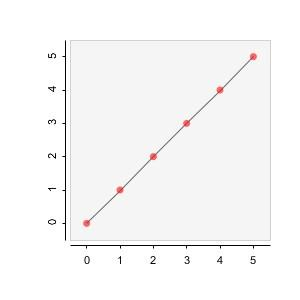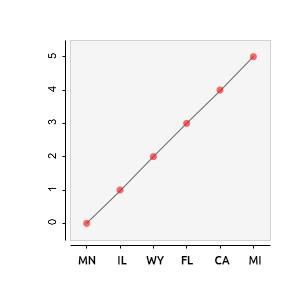#### Howdy, Stranger!

We are about to switch to a new forum software. Until then we have removed the registration on this forum.

# grafica replacing axis ticks with new strings

edited March 2017

Is there a way to change to replace the label ticks for the x/y axis with a string instead of the associated number? For example let's look at this code:

``````import grafica.*;

GPlot plot;

GPointsArray myArray = new GPointsArray(0);

void setup() {
size(500, 500);

plot = new GPlot(this, 0, 0, 500, 500);

plot.setPoints(myArray);
plot.defaultDraw();
}
``````

It creates this graph:I need it to appear like this by replacing each tick with a new string:Tagged:

• according to this:

https://github.com/jagracar/grafica/blob/master/src/grafica/GPointsArray.java#L171

there's another GPointsArray.add() call that allows a label.

``````public void add(float x, float y, String label) {
....
``````
• edited March 2017

I tried doing this but it still only shows numbers when drawing the graph:

``````myArray.add(0, 0, "MN");
``````
• Aren't GPointsArray labels meant to be the labels for individual data points? I think the documentation for axis labels is here:

...or maybe not. Now that I glance over it, I'm not sure.

• ah, ok.

i based my answer on a couple of minutes of searching on my phone, specifically the Gaussian bit of this example:

http://jagracar.com/sketches/multiplePlots.php

i figured the H labels at the bottom there were similar to the requirements

which is line 85 here https://github.com/jagracar/grafica.js/blob/master/examples/multiplePlots.js#L85

``````points3[i] = new GPoint(i + 0.5 - gaussianStack.length / 2, gaussianStack[i] / gaussianCounter, "H" + i);
``````

or line 217

https://github.com/jagracar/grafica.js/blob/master/examples/multiplePlots.js#L217

``````points3[i] = new GPoint(i + 0.5 - gaussianStack.length / 2, gaussianStack[i] / gaussianCounter, "H" + i);
``````

BUT that's javascript.

the relevant java versions are here (Lines 83 and 211):

https://github.com/jagracar/grafica/blob/master/examples/MultiplePlots/MultiplePlots.pde

and look like this:

``````points3.add(i + 1 - gaussianStack.length/2.0, gaussianStack[i]/gaussianCounter, "H" + i);
``````

so i don't really understand why that's not working based on the 5 minutes i've looked at it 8)

maybe i should try running this...

• ok, i have had a play

replace the plot.defaultDraw() with this, which draws all the bits

``````  plot.beginDraw();
plot.drawBackground();
plot.drawBox();
plot.drawYAxis();
plot.drawTitle();
plot.getHistogram().setDrawLabels(true);
plot.drawHistograms();
plot.endDraw();
``````

and you get labels. for the histogram.

the same thing doesn't happen when you use drawPoints(). tbh, labels make more sense for histograms than for points...

• i think the points equiv is

https://github.com/jagracar/grafica/blob/master/src/grafica/GPlot.java#L2764

``````activatePointLabels(int button, int keyModifier)
``````

which, as jeremy points out, labels the point (on mouseover), not the axis

• Interesting solution, @koogs ! For some reason when I take the OP sketch and replace `plot.defaultDraw()` with your eight lines, above, as per your instructions, I get a `NullPointerException` on your line:

``````plot.getHistogram().setDrawLabels(true);
``````

Is there a bit more setup needed?

(That's on Processing 3.2.3 with grafica 1.7.0.)

• Oh there might've been a line added to the graph setup (which was separate from the drawing code). But I can't remember what it was and I've deleted the sketch now. It was based on the Java example linked above.

• Probably this

``````plot.startHistograms(GPlot.VERTICAL);
``````
• That worked, thanks. A histogram, as you said, not a line graph as OP requested -- but it has category axis labels, and the bars probably make more sense for categorical data.

``````import grafica.*;

GPlot plot;
GPointsArray myArray = new GPointsArray(0);

void setup() {
size(500, 500);

// create plot
plot = new GPlot(this, 0, 0, 500, 500);
plot.startHistograms(GPlot.VERTICAL);

plot.setPoints(myArray);

// draw plot
plot.beginDraw();
plot.drawBackground();
plot.drawBox();
plot.drawYAxis();
plot.drawTitle();
plot.getHistogram().setDrawLabels(true);
plot.drawHistograms();
plot.endDraw();
}
``````

Thanks for your responses. I was specifically looking for labels for line graphs though. Fortunatly I found it! Check out setTickLabels()

Solution to my original example:

``````plot.setPoints(myArray);
String[] newLabels = {"MN", "IL", "WY", "FL", "CA", "MI"};
plot.getXAxis().setTickLabels(newLabels);
plot.defaultDraw();
``````
• Thanks for sharing that solution, @Joey.

For the curious: none of the grafica example sketches demonstrate the use of `GAxis.setTickLabels()`, but you can find the method here: